# JPWO2013175951A1 - Receiver, Doppler frequency calculation method, and computer program - Google Patents

## Info

Publication number
JPWO2013175951A1
JPWO2013175951A1 JP2013062704A JP2014516741A JPWO2013175951A1 JP WO2013175951 A1 JPWO2013175951 A1 JP WO2013175951A1 JP 2013062704 A JP2013062704 A JP 2013062704A JP 2014516741 A JP2014516741 A JP 2014516741A JP WO2013175951 A1 JPWO2013175951 A1 JP WO2013175951A1
Authority
JP
Japan
Prior art keywords
doppler frequency
channel estimation
step
unit
estimation value
Prior art date
Legal status (The legal status is an assumption and is not a legal conclusion. Google has not performed a legal analysis and makes no representation as to the accuracy of the status listed.)
Pending
Application number
JP2013062704A
Other languages
Japanese (ja)
Inventor

Original Assignee

Priority date (The priority date is an assumption and is not a legal conclusion. Google has not performed a legal analysis and makes no representation as to the accuracy of the date listed.)
Filing date
Publication date
Priority to JP2012117452 priority Critical
Priority to JP2012117452 priority
Application filed by 日本電気株式会社, 株式会社Ｎｔｔドコモ filed Critical 日本電気株式会社
Priority to JP2013062704A priority patent/JPWO2013175951A1/en
Priority to PCT/JP2013/062704 priority patent/WO2013175951A1/en
Publication of JPWO2013175951A1 publication Critical patent/JPWO2013175951A1/en
Application status is Pending legal-status Critical

## Images

•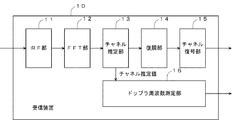•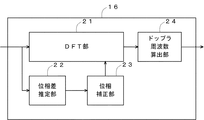•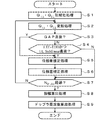•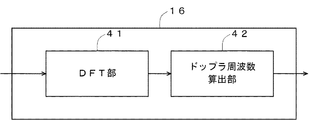•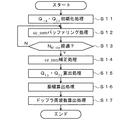## Classifications

• HELECTRICITY
• H04ELECTRIC COMMUNICATION TECHNIQUE
• H04LTRANSMISSION OF DIGITAL INFORMATION, e.g. TELEGRAPHIC COMMUNICATION
• H04L27/00Modulated-carrier systems
• H04L27/26Systems using multi-frequency codes
• H04L27/2601Multicarrier modulation systems
• H04L27/2647Arrangements specific to the receiver
• H04L27/2655Synchronisation arrangements
• H04L27/2657Carrier synchronisation
• HELECTRICITY
• H04ELECTRIC COMMUNICATION TECHNIQUE
• H04LTRANSMISSION OF DIGITAL INFORMATION, e.g. TELEGRAPHIC COMMUNICATION
• H04L27/00Modulated-carrier systems
• H04L27/26Systems using multi-frequency codes
• H04L27/2601Multicarrier modulation systems
• H04L27/2647Arrangements specific to the receiver
• H04L27/2655Synchronisation arrangements
• H04L27/2668Details of algorithms
• H04L27/2669Details of algorithms characterised by the domain of operation
• H04L27/2672Frequency domain
• HELECTRICITY
• H04ELECTRIC COMMUNICATION TECHNIQUE
• H04LTRANSMISSION OF DIGITAL INFORMATION, e.g. TELEGRAPHIC COMMUNICATION
• H04L27/00Modulated-carrier systems
• H04L27/26Systems using multi-frequency codes
• H04L27/2601Multicarrier modulation systems
• H04L27/2647Arrangements specific to the receiver
• H04L27/2655Synchronisation arrangements
• H04L27/2668Details of algorithms
• H04L27/2673Details of algorithms characterised by synchronisation parameters
• H04L27/2675Pilot or known symbols
• HELECTRICITY
• H04ELECTRIC COMMUNICATION TECHNIQUE
• H04LTRANSMISSION OF DIGITAL INFORMATION, e.g. TELEGRAPHIC COMMUNICATION
• H04L27/00Modulated-carrier systems
• H04L27/26Systems using multi-frequency codes
• H04L27/2601Multicarrier modulation systems
• H04L27/2647Arrangements specific to the receiver
• H04L27/2655Synchronisation arrangements
• H04L27/2689Link with other circuits, i.e. special connections between synchronisation arrangements and other circuits for achieving synchronisation
• H04L27/2695Link with other circuits, i.e. special connections between synchronisation arrangements and other circuits for achieving synchronisation with channel estimation, e.g. determination of delay spread, derivative or peak tracking

## Abstract

Even when the phase of the channel estimation value in the time direction may be discontinuous, the Doppler frequency is correctly calculated. A receiving unit (RF unit 11) that receives a transmission signal transmitted from a transmission device, a conversion unit (FFT unit 12) that converts the transmission signal received by the receiving unit into a frequency domain signal, and a conversion An estimation unit (channel estimation unit 13) that executes channel estimation processing based on a frequency domain signal obtained by the unit, and a calculation unit (Doppler frequency) that calculates a Doppler frequency based on the channel estimation value estimated by the estimation unit And the calculation means, when there is a possibility that the phase of the channel estimation value in the time direction is discontinuous, after performing correction processing on the data in the middle of calculating the Doppler frequency The Doppler frequency is calculated.

## Description

The present invention relates to a receiving apparatus, a Doppler frequency calculation method, and a computer program.

In wireless communication systems such as LTE (Long Term Evolution) standardized by 3GPP (3rd Generation Partnership Project) in recent years, various performances can be improved by switching control according to the Doppler frequency estimated by the receiving apparatus. Technology has been developed.

For example, Patent Document 1 discloses a technique for ensuring transmission quality of data and subscriber capacity in a cell by performing transmission power control based on a Doppler frequency. In order to perform such control, it is necessary to accurately estimate the Doppler frequency. As a method for estimating the Doppler frequency, for example, there is a method of performing a discrete Fourier transform (DFT) on a channel estimation value.

Patent Document 2 discloses a technique for estimating an optimal Doppler frequency after performing DFT on channel estimation values in each of the time direction and the frequency direction to determine whether the radio wave environment is within the line of sight.

Patent Document 3 discloses a technique for reducing the amount of calculation in a receiving apparatus by performing DFT using Goertzel Algorithm.

Japanese Patent No. 3430986 JP 2010-258935 A US Patent Application Publication No. US2011 / 020073 Specification

By the way, for example, in the case of LTE, the phase synchronization circuit (PLL: Phase-Locked Loop) is re-executed immediately after GAP between uplink and downlink communication, immediately after UL (Uplink) Subframe in LTE-TDD (Time Division Duplex), and the like. When activated, the phase of the channel estimation value in the time direction may become discontinuous. When estimating the Doppler frequency by performing DFT in the time direction, if the phase of the channel estimation value in the time direction becomes discontinuous during the Doppler frequency estimation period, the Doppler frequency cannot be estimated accurately. . In the above-described Patent Documents 2 to 3, there is a problem that the Doppler frequency cannot be accurately estimated in such a case.

The present invention has been made in view of the problems as described above, and is capable of correctly calculating the Doppler frequency even when the phase of the channel estimation value in the time direction may be discontinuous. An object is to provide an apparatus, a Doppler frequency calculation method, and a computer program.

In order to solve the above-described problem, according to a first aspect of the present invention, in a receiving device that performs wireless communication with a transmitting device, a receiving unit that receives a transmission signal transmitted from the transmitting device; Conversion means for converting the transmission signal received by the means into a frequency domain signal, estimation means for executing a channel estimation process based on the frequency domain signal obtained by the conversion means, and estimation by the estimation means Calculating means for calculating the Doppler frequency based on the channel estimation value, and the calculating means obtains the Doppler frequency when the phase of the channel estimation value in the time direction may be discontinuous. There is provided a receiving apparatus characterized by calculating a Doppler frequency after performing correction processing on data being calculated.

According to a second aspect of the present invention, in a Doppler frequency calculation method for a receiving apparatus that performs wireless communication with a transmitting apparatus, a receiving step for receiving a transmission signal transmitted from the transmitting apparatus, and reception at the receiving means A conversion step of converting the transmitted signal into a frequency domain signal, an estimation step of executing a channel estimation process based on the frequency domain signal obtained in the conversion step, and a channel estimated in the estimation step A calculation step of calculating a Doppler frequency based on the estimation value, and the calculation step is in the middle of calculating the Doppler frequency when the phase of the channel estimation value in the time direction may be discontinuous. A Doppler characterized by calculating a Doppler frequency after performing correction processing on the data. The wave number calculation method is provided.

According to a third aspect of the present invention, by installing in a processor of a receiving device that performs wireless communication with a transmitting device, the processor receives a transmission signal transmitted from the transmitting device; A conversion step of converting the transmission signal received by the receiving means into a frequency domain signal; an estimation step of performing a channel estimation process based on the frequency domain signal obtained in the conversion step; and the estimation step A calculation step of calculating a Doppler frequency based on the channel estimation value estimated in Step 1, and in the calculation step, if there is a possibility that the phase of the channel estimation value in the time direction is discontinuous, the Doppler frequency After applying correction to the data that is being calculated, calculate the Doppler frequency. Computer programs for causing the processing to be executed is provided.

According to the present invention, it is possible to provide a receiver, a Doppler frequency calculation method, and a computer program capable of correctly calculating a Doppler frequency even when the phase of a channel estimation value in the time direction may be discontinuous. It becomes possible to do.

It is a block diagram which shows the structural example of 1st Embodiment of this invention. It is a block diagram which shows the detailed structural example of the Doppler frequency measurement part shown in FIG. It is a flowchart for demonstrating operation | movement of 1st Embodiment. It is a block diagram which shows the structural example of the Doppler frequency measurement part which concerns on 2nd Embodiment of this invention. It is a flowchart for demonstrating operation | movement of 2nd Embodiment.

Next, an embodiment of the present invention will be described.

(A) Description of First Embodiment FIG. 1 is a block diagram showing a configuration example of the first embodiment of the present invention. As shown in FIG. 1, the receiving apparatus 10 according to the first embodiment of the present invention includes an RF (Radio Frequency) unit 11, an FFT (Fast Fourier Transform) unit 12, a channel estimation unit 13, a demodulation unit 14, and a channel decoding. Unit 15 and Doppler frequency measurement unit 16 and operate based on the LTE standard standardized in 3GPP.

Here, the RF unit 11 receives a radio wave transmitted from a base station (not shown), converts the radio wave into a digital signal by A / D (Analog to Digital) conversion, and outputs the digital signal to the FFT unit 12. The FFT unit 12 performs a Fourier transform on the digital signal supplied from the RF unit 11, thereby converting the digital signal into a frequency domain signal (I, Q signal), and outputs the signal to the channel estimation unit 13.

The channel estimation unit 13 estimates a downlink channel state from a reference signal (RS: Reference Signal) that is a known signal mapped in advance on a frequency resource, and uses the estimation result as a channel estimation value to determine the demodulation unit 14 and the Doppler frequency. Output to the measurement unit 16. The demodulation unit 14 refers to the channel estimation value output from the channel estimation unit 13, demodulates the I and Q information into likelihood information, and outputs the likelihood information to the channel decoding unit 15. The channel decoding unit 15 performs error correction decoding and error detection on the likelihood information output from the demodulation unit 14, and outputs the obtained information to a higher layer device. The Doppler frequency measurement unit 16 refers to the channel estimation value supplied from the channel estimation unit 13, measures the Doppler frequency, and outputs the obtained measurement result to the upper layer device. The upper layer apparatus performs, for example, transmission power control based on the Doppler frequency.

FIG. 2 is a block diagram showing a detailed configuration example of the Doppler frequency measurement unit 16 shown in FIG. As illustrated in FIG. 2, the Doppler frequency measurement unit 16 includes a DFT unit 21, a phase difference estimation unit 22, a phase correction unit 23, and a Doppler frequency calculation unit 24.

Here, the DFT unit 21 performs DFT based on the channel estimation value estimated by the channel estimation unit 13 and calculates a delay spread that is an index indicating how much the delay amount is from the average. The phase difference estimation unit 22 determines whether the phase may be discontinuous (immediately after GAP, immediately after UL Subframe at LTE TDD, etc.), and may be discontinuous. If this is the case, the phase difference is estimated from the channel estimation value. The phase correction unit 23 executes the phase correction process based on the phase difference estimated by the phase difference estimation unit 22 when the phase may be discontinuous. The Doppler frequency calculation unit 24 determines whether or not a predetermined slot has elapsed from the start of measurement. If the predetermined slot has elapsed, the Doppler frequency calculation unit 24 calculates the Doppler frequency and supplies it to the upper layer apparatus.

Next, the operation of the first embodiment will be described with reference to the flowchart shown in FIG. When the flowchart shown in FIG. 3 is started, the following steps are executed.

In step S1, when the first slot is input, the DFT unit 21 executes an initialization process based on the following equation (1).

Here, h fd indicates a channel estimation value used for Doppler frequency measurement, a indicates the order of receiving antennas, b indicates the order of transmitting antennas, n indicates a slot number, and i is an index of RS (Reference Signal). N tx represents the number of transmission antennas, N ave_RS represents the number of RSs used for Doppler frequency measurement, and N calc_bins represents the number of DFT bins used for Doppler frequency measurement. Re {} represents a real part in parentheses, and Im {} represents an imaginary part in parentheses.

In step S2, the DFT unit 21 calculates coefficients Q1n , k, 0 · Q2n , k, 0 · Q1n , k, 1 · Q2n , k, 1 for the slots after the first time as follows: Update according to 2).

However, DFT_COEFF k is a DFT coefficient and is calculated | required by the following formula | equation (3). N fd_slots indicates the number of slots used for Doppler frequency measurement.

In step S3, the phase difference estimator 22 determines whether or not it is immediately after the GAP that may cause the phase to be discontinuous. If it is determined that the phase is applicable (step S3: Yes), the process proceeds to step S5. In other cases (step S3: No), the process proceeds to step S4. Specifically, if it is immediately after GAP between upstream and downstream communications, it is determined Yes and the process proceeds to step S5.

In step S4, the phase difference estimation unit 22 determines whether or not it corresponds immediately after UL Subframe in LTE-TDD in which the phase may also be discontinuous, and determines that it corresponds (step S4: Yes). The process proceeds to step S5, and otherwise (step S4: No), the process proceeds to step S7.

In step S5, the phase difference estimation unit 22 estimates the phase difference φ diff from the channel estimation value based on the following equation (4).

In step S <b> 6, the phase correction unit 23 calculates the phase based on the following equation (5) based on the phase difference φ diff estimated by the phase difference estimation unit 22 in the case where the phase may be discontinuous. The correction is executed, and the correction result is output to the Doppler frequency calculation unit 24.

In step S7, the Doppler frequency calculation unit 24 determines whether or not N fd_slots has elapsed from the start of measurement. If it is determined that it has elapsed (step S7: Yes), the process proceeds to step S8, and otherwise ( In step S7: No), the process returns to step S2 and the same process as described above is repeated.

In step S8, the Doppler frequency calculation unit 24 calculates the amplitude based on the following equation (6).

In step S9, the Doppler frequency calculation unit 24, when determining the DFT peak as shown in the following equation (7), DFT k, a maximum of k that satisfies j> DFT peak × X fd and k edge, below The Doppler frequency Fd is calculated based on the equation (8).

However, X fd is a threshold coefficient for Doppler frequency calculation, and Fd_COEFF is calculated by the following equation (9).

As described above, according to the first embodiment, when there is a possibility that the phase of the channel estimation value in the time direction may be discontinuous, a process for estimating and correcting the phase difference is added. It is possible to calculate a correct Doppler frequency.

(B) Description of Second Embodiment FIG. 4 is a diagram illustrating a configuration example of a Doppler frequency measurement unit 16 according to a second embodiment of the present invention. The configuration other than the Doppler frequency measurement unit 16 is the same as that in FIG. As illustrated in FIG. 4, the Doppler frequency measurement unit 16 according to the second embodiment includes a DFT unit 41 and a Doppler frequency calculation unit 42.

Here, the DFT unit 41 performs DFT based on the channel estimation value estimated by the channel estimation unit 13 and calculates the delay spread described above. The Doppler frequency calculation unit 42 determines whether or not a predetermined slot has elapsed from the start of measurement. If the predetermined slot has elapsed, the Doppler frequency calculation unit 42 calculates the Doppler frequency and supplies it to the upper layer apparatus. In the first embodiment, the phase difference is estimated and corrected. However, in the second embodiment, the Doppler frequency is obtained by performing DFT processing on the power of the channel estimation value.

Next, the operation of the second embodiment shown in FIG. 4 will be described with reference to the flowchart shown in FIG.

In step S11, the DFT processing unit 41 initializes the initial slot based on the following equation (10).

Note that the various parameters in Expression (10) are the same as in Expression (1), and thus detailed description thereof is omitted.

In step S12, the DFT processing unit 41 performs processing of buffering ce_sum for the first and subsequent slots based on the following equation (11).

In step S13, the DFT processing unit 41 determines whether or not N fd_slots has elapsed since the start of measurement. If it is determined that N fd_slots has elapsed (step S13: Yes), the process proceeds to step S14, and otherwise (step S13). In step S13: No), the process returns to step S12 to continue the buffering process. N fd_slots indicates the number of slots used for Doppler frequency measurement, as in step S7 described above.

In step S14, the DFT processing unit 41 performs a process of correcting ce_sum based on the following equation (12) in order to remove the direct current component.

In step S15, the DFT processing unit 41 executes processing for obtaining the coefficients Q1 n, k · Q2 n, k based on the following equation (13), and these obtained coefficients Q1 n, k · Q2 n are obtained. , K are output to the Doppler frequency calculation unit 42.

In step S <b> 16, the Doppler frequency calculation unit 42 calculates the amplitude based on the following equation (14).

In step S17, the Doppler frequency calculation unit 42, when determining the DFT peak by the following equation (15), DFT k> DFT peak × the maximum k satisfying X Fd and k edge, the following equation (16 ) To calculate the Doppler frequency Fd. The Doppler frequency calculated in this way is output to a higher layer device. The upper layer apparatus performs, for example, transmission power control based on the Doppler frequency.

As described above, according to the second embodiment, since the power of the channel estimation value is DFT-processed, there is a possibility that the phase of the channel estimation value in the time direction may be discontinuous. However, it is possible to calculate a correct Doppler frequency.

(C) Description of Modified Embodiment It goes without saying that the above embodiment is merely an example, and the present invention is not limited to the case described above. For example, in the above embodiment, the LTE standardized in 3GPP has been described as an example, but the present invention can be applied to other communication systems.

Further, in each of the above embodiments, the case where the present invention is applied to a mobile phone has been described as an example. However, other than this, for example, PHS (Personal Handy-phone System) or DECT (Digital Enhanced Cordless Communications) The present invention can also be applied to a wireless LAN (Local Area Network) or the like.

Furthermore, at least a part of the operation of each unit in the above embodiments can be realized by a computer including a processor, a RAM (random access memory), a ROM (read only memory), and the like. In this case, by installing the present invention in a processor of a receiving device that performs wireless communication with the transmitting device, the processor receives the transmission signal transmitted from the transmitting device, and the receiving means A conversion step for converting the transmission signal received in the step into a frequency domain signal, an estimation step for performing a channel estimation process based on the frequency domain signal obtained in the conversion step, and an estimation step in the estimation step. A calculation step of calculating a Doppler frequency based on the channel estimation value, and calculating a Doppler frequency when the phase of the channel estimation value in the time direction may be discontinuous in the calculation step. A process for calculating the Doppler frequency after correction processing is performed on intermediate data. It can also be implemented as a computer program for execution.

DESCRIPTION OF SYMBOLS 10 Receiver 11 RF part 12 FFT part 13 Channel estimation part 14 Demodulation part 15 Channel decoding part 16 Doppler frequency measurement part 21 DFT part 22 Phase difference estimation part 23 Phase correction part 24 Doppler frequency calculation part 41 DFT part 42 Doppler frequency calculation part

## Claims (6)

1. In a receiving device that performs wireless communication with a transmitting device,
Receiving means for receiving a transmission signal transmitted from the transmission device;
Converting means for converting the transmission signal received by the receiving means into a signal in a frequency domain;
Estimating means for performing channel estimation processing based on the signal in the frequency domain obtained by the converting means;
Calculation means for calculating a Doppler frequency based on the channel estimation value estimated by the estimation means,
If the phase of the channel estimation value in the time direction is likely to be discontinuous, the calculation means calculates the Doppler frequency after performing correction processing on the data in the middle of calculating the Doppler frequency.
A receiving apparatus.
2.   The calculation means estimates a phase difference from the channel estimation value when there is a possibility that the phase of the channel estimation value is discontinuous, and corrects the data being calculated based on the estimated phase difference. The receiver according to claim 1, wherein the Doppler frequency is calculated.
3.   The calculation means performs the correction process on the assumption that the phase discontinuity may occur immediately after GAP between uplink and downlink communication or immediately after UL Subframe in LTE-TDD. The receiving device according to claim 2.
4.   The calculation means calculates the Doppler frequency by performing a DFT process on the power of the channel estimation value and executing a correction process for removing a DC component from the obtained processing result. The receiving device according to 1.
5. In the Doppler frequency calculation method of the receiving device that performs wireless communication with the transmitting device,
A reception step of receiving a transmission signal transmitted from the transmission device;
A converting step of converting the transmission signal received by the receiving means into a signal in a frequency domain;
An estimation step for performing a channel estimation process based on the frequency domain signal obtained in the conversion step;
A calculation step of calculating a Doppler frequency based on the channel estimation value estimated in the estimation step,
In the calculation step, when there is a possibility that the phase of the channel estimation value in the time direction is discontinuous, the Doppler frequency is calculated after performing correction processing on the data in the middle of calculating the Doppler frequency.
A Doppler frequency calculation method for a receiving apparatus.
6.   A reception step of receiving a transmission signal transmitted from the transmission apparatus by the processor of the reception apparatus that performs wireless communication with the transmission apparatus, and the transmission signal received by the reception unit. Is converted into a frequency domain signal, an estimation step is performed based on the frequency domain signal obtained in the conversion step, and a channel estimation value estimated in the estimation step is based on A calculation step for calculating a Doppler frequency, and in the calculation step, if there is a possibility that the phase of the channel estimation value in the time direction is discontinuous, correction is performed for data in the middle of calculating the Doppler frequency. After performing the processing, let's execute the processing to calculate the Doppler frequency. Computer programs that butterflies.
JP2013062704A 2012-05-23 2013-05-01 Receiver, Doppler frequency calculation method, and computer program Pending JPWO2013175951A1 (en)

## Priority Applications (4)

Application Number Priority Date Filing Date Title
JP2012117452 2012-05-23
JP2012117452 2012-05-23
JP2013062704A JPWO2013175951A1 (en) 2012-05-23 2013-05-01 Receiver, Doppler frequency calculation method, and computer program
PCT/JP2013/062704 WO2013175951A1 (en) 2012-05-23 2013-05-01 Receiving device, doppler frequency computation method, and computer program

## Applications Claiming Priority (1)

Application Number Priority Date Filing Date Title
JP2013062704A JPWO2013175951A1 (en) 2012-05-23 2013-05-01 Receiver, Doppler frequency calculation method, and computer program

## Publications (1)

Publication Number Publication Date
JPWO2013175951A1 true JPWO2013175951A1 (en) 2016-01-12

# Family

## Family Applications (1)

Application Number Title Priority Date Filing Date
JP2013062704A Pending JPWO2013175951A1 (en) 2012-05-23 2013-05-01 Receiver, Doppler frequency calculation method, and computer program

## Country Status (2)

JP (1) JPWO2013175951A1 (en)
WO (1) WO2013175951A1 (en)

## Family Cites Families (4)

* Cited by examiner, † Cited by third party
Publication number Priority date Publication date Assignee Title
JP5109878B2 (en) * 2008-09-03 2012-12-26 富士通株式会社 Demodulator
US8599977B2 (en) * 2008-10-24 2013-12-03 Nec Corporation Device and method for estimating doppler spread in a mobile communications terminal
JP5344984B2 (en) * 2009-04-28 2013-11-20 三菱電機株式会社 Moving speed detection device and moving speed detection method
GB2470753A (en) * 2009-06-03 2010-12-08 Sony Corp Receiver and method for processing pilot data in an ofdm system

## Also Published As

Publication number Publication date
WO2013175951A1 (en) 2013-11-28

## Similar Documents

Publication Publication Date Title
US9544919B2 (en) Method and apparatus for transmitting and receiving uplink random access channel slot in a wireless communication system using beamforming
US9173217B2 (en) Base station calibration
JP5351926B2 (en) Wireless communication device
ES2478011T3 (en) Measures of time synchronization difference in OFDM systems
KR100865938B1 (en) Apparatus for Estimating and Compensating Carrier Frequency Offset and Data Receiving Method in Receiver of Wireless Communication System
EP2456078B1 (en) Method and device for frequency deviation pre-correction
US8929191B2 (en) Base station device for wireless communication of OFDM signal including a synchronization control unit
EP2987370B1 (en) Secondary cell synchronization for carrier aggregation
KR101314314B1 (en) Method and Apparatus for Uplink Timing Synchronization with Ranging Signal in TDD Mobile Communication System
JP6065071B2 (en) Communication device, reference signal receiving method, and integrated circuit
US8213527B2 (en) Channel variation detection of wireless channel for adaptive transmission schemes
JP5803795B2 (en) Receiver, frequency deviation calculation method, and computer program
US7599453B2 (en) Doppler spread estimation for OFDM systems
JP4924201B2 (en) Reception quality measuring apparatus and reception quality measuring method
RU2417541C2 (en) Single-packet detection for wireless communication network
US20110243104A1 (en) Method and system for tracking timing drift in multiple frequency hypothesis testing
US8982797B2 (en) Wireless communication apparatus with resource allocation to users performing array combining using array combining weights